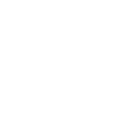×4th Chapter

### 8th Mathematics Urdu Medium Chapter 4 Test

Here you can prepare 8th Mathematics Urdu Medium Chapter 4 Test. Click the button for 8th Mathematics Urdu Medium Chapter 4 100% free full practice test.

## 8th Class Mathematics Chapter 4 MCQ Test With Answer for Mathematics chapter 4

8th Class Mathematics Ch. 4 Online Test### 8th Class Mathematics Chapter 4 MCQ Test With Answer for Mathematics chapter 4

1 A composite 3D figure is a three-dimentional figure mae up of basic.
• A. 2D figures
• B. 3D figures
• C. Pyramid
• D. 2D and 3D figures
2 {0,1,2,3,.............} IS A SET OF.
• A. N
• B. W
• C. Z
• D. Q
3 The sum of interior angles of a traingle is
• A. 60 o
• B. 90 o
• C. 180 o
• D. 360 o
4 If 3y-x = 4, find the value of y , when x = 2
• A. 4
• B. 2
• C. 3
• D. 1/2
5 The types of transformation are.
• A. 3
• B. 4
• C. 5
• D. 6
6 Cube of an even number is.
• A. Even
• B. Odd
• C. Zero
• D. One
7 Cube of an odd number is.
• A. Even
• B. Odd
• C. Zero
• D. One
8 Triangle has angles.
• A. 3
• B. 2
• C. 4
• D. 1
9 If the object and figures have exactly the same shapes and sizes then they are called.
• A. Similar objects
• B. Congurent object
• C. Transformation
• D. Rotation
10 The difference betwene approximated value and actual accurate value is caled
• A. Round
• B. Significant figure
• C. Appoximation error
• D. Estimated value

Sort By:
X

to continue to ilmkidunya.comFill the form. Our admission consultants will call you with admission options.

X

to continue to ilmkidunya.com

X

to continue to ilmkidunya.com

X